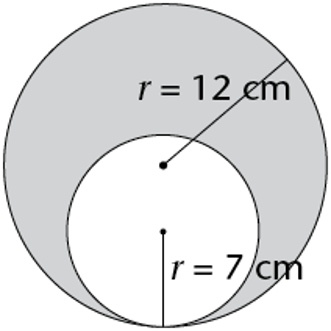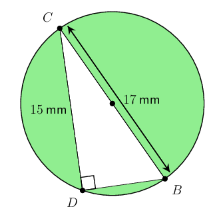Home Practice
For learners and parents For teachers and schools
Textbooks
Full catalogue
Pricing SupportLog in

We think you are located in United States. Is this correct?

# Solving problems involving area and perimeter

## Worked example 16.19: Solving problems involving areas of circles

Calculate the area of the shaded region. Use $$\pi = \text{3,14}$$ for your calculations.### Calculate the area of the larger circle.

\begin{align} \text{Area of larger circle } &= \pi r^2 \\ &= \text{3,14}(12)^2 \\ &= \text{3,14}(144) \\ &= \text{452,16} \text{ cm}^2 \\ \end{align}

### Calculate the area of the smaller circle.

\begin{align} \text{Area of smaller circle } &= \pi r^2 \\ &= \text{3,14}(7)^2 \\ &= \text{3,14}(49) \\ &= \text{153,86}\text{ cm}^2 \end{align}

### Find the area of the shaded region.

\begin{align} \text{Area of the shaded region } &= \text{(area of larger circle)} − \text{(area of smaller circle)} \\ &= \text{452,16} \text{ cm}^2− \text{153,86} \text{ cm}^2 \\ &= \text{298,3} \text{ cm}^2 \end{align}

$$\text{Area of shaded region } = \text{298,3} \text{ cm}^2$$.

## Worked example 16.20: Solving problems involving areas of circles and triangles

The diagram shows a circle and a right-angled triangle drawn within the circle. $$BC$$ is a diameter of the circle. $$BC = 17 \text{ mm}$$ and $$CD = 15 \text{ mm}$$.

Determine the area of the shaded region. Use $$\pi = \frac{22}{7}$$, and give your answer correct to two decimal places.### Calculate the area of the circle.

The diameter of the circle is $$BC = \text{17} \text{ mm}$$, so we can divide by $$2$$ to get the radius:

$r = \frac{d}{2} = \frac{17}{2} = \text{8,5}$ \begin{align} \text{Area of circle } &= \pi r^2 \\ &= \pi (\text{8,5})^2 \\ &= \frac{22}{7} \times \text{72,25} \\ &= \text{227,07} \text{ mm}^2 \end{align}

### Calculate the area of the right-angled triangle.

$$CD = \text{15} \text{ mm}$$ is the perpendicular height of the triangle. Since this is a right-angled triangle, we can use the theorem of Pythagoras to determine the length of the base of the triangle $$BD$$.

In $$\triangle BCD$$:

\begin{align} BD^2 + CD^2 &= BC^2 \text{ (Pythagoras)} \\ BD^2 + 15^2 &= 17^2 \\ BD^2 &= 289 − 225 \\ BD^2 &= 64 \\ \therefore BD &= 8 \end{align}

So base $$BD = 8 \text{ mm}$$

We can now calculate the area of the triangle $$\triangle BCD$$:

\begin{align} \text{Area } \triangle BCD &= \frac{1}{2}(b \times h) \\ &= \frac{1}{2}(8 \times 15) \\ &= \frac{1}{2}(120) \\ &= 60 \text{ mm}^2 \end{align}

### Calculate the area of the shaded region.

\begin{align} \text{Area of the shaded region } &= \text{(area of circle)} − \text{(area of triangle)} \\ &= \text{227,07} \text{ mm}^2 – 60 \text{ mm}^2 \\ &= \text{167,07} \text{ mm}^2 \end{align}

Area of the shaded region $$= \text{167,07} \text{ mm}^2$$.